# Maximum Efficiency for Class B Power Amplifier

## Maximum Efficiency for Class B Power Amplifier

We have already seen in our previous article about class B  power amplifier that a push-pull circuit uses two transistors working in class B operation .

For class B operation, the Q – point is located at cut-off on both d.c. and a.c. load lines .

For maximum signal operation, the two transistors in class B amplifier are alternately driven from cut-off to saturation . This is shown in fig.1 .Fig.1

It is clear that a.c. output voltage has a peak value of  VCE  and a.c. output current has a peak value of IC(sat) .

The same information is conveyed through the a.c. load line for the circuit as shown in fig.2 .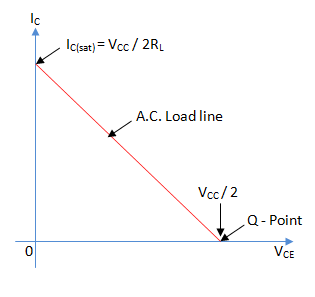Fig. 2Since the two transistors are identical, half the supply voltage is dropped across each transistor’s collector-emitter terminals.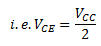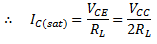Maximum average a.c. output  power Po(max)  is

Po(max)  = Product of r.m.s. values of a.c. output voltage and a.c. output current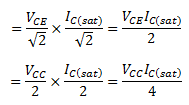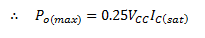The input d.c. power from the supply VCC is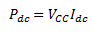Where Idc  is the average current drawn from the supply VCC .

Since, the transistor is on for alternating half-cycles, it effectively acts as a half-wave rectifier .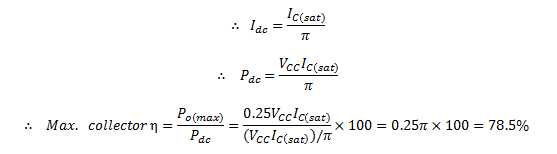Thus, the maximum collector efficiency of class B power amplifier is 78.5% .